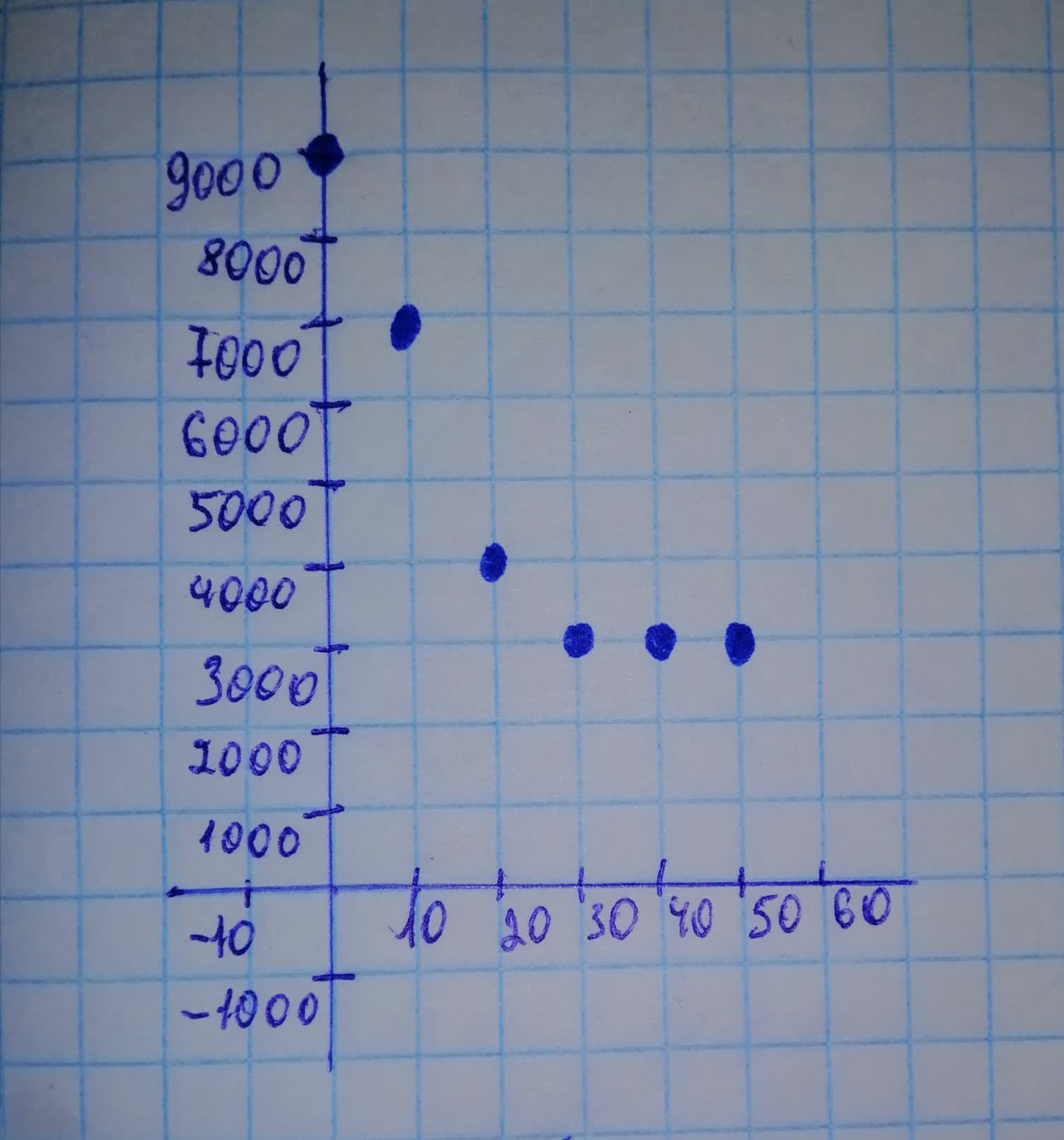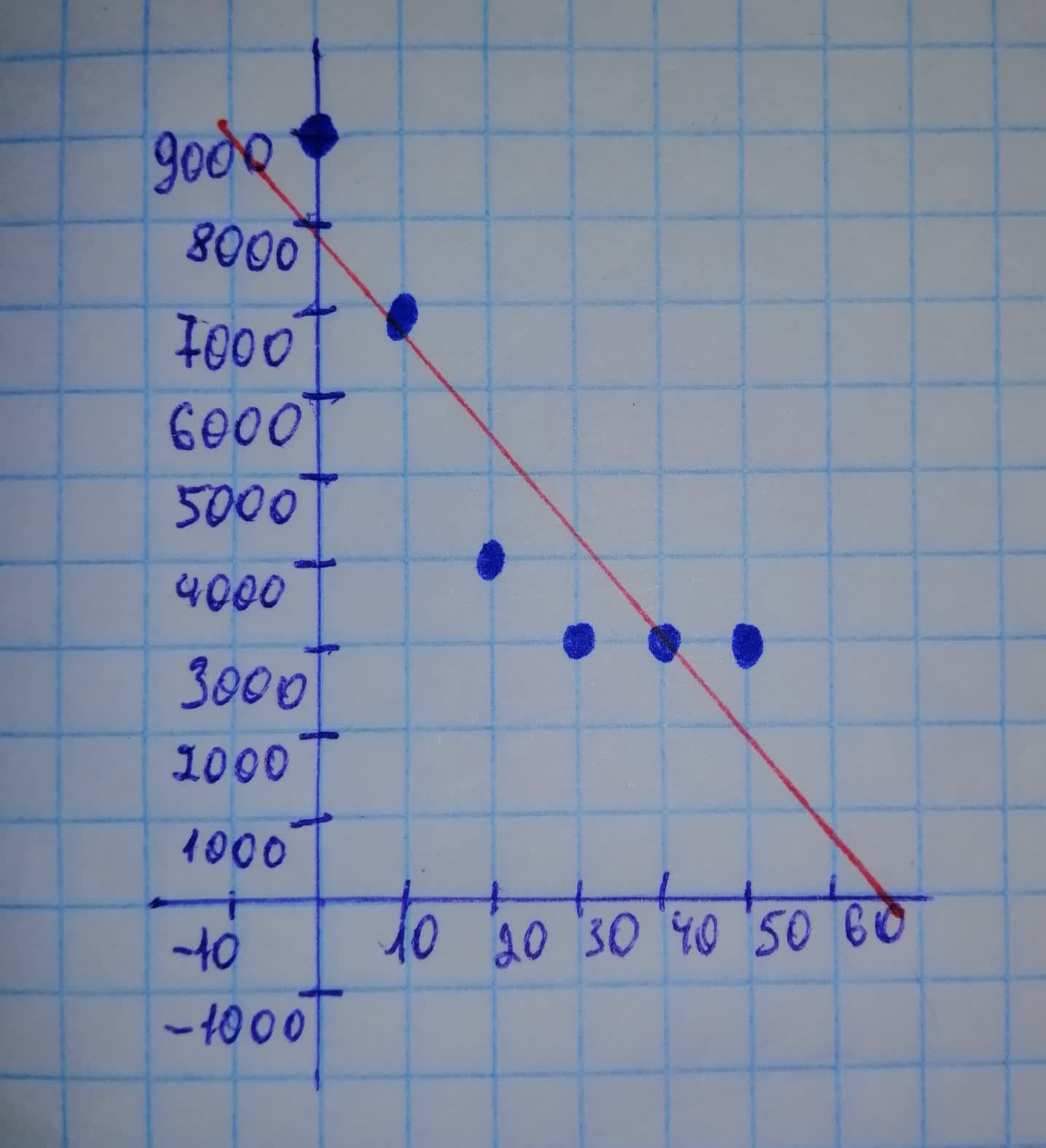# Make a scatterplot for the data below on the number of people working on farms iosi4a2nxk 2021-11-16 Answered
Make a scatterplot for the data below on the number of people working on farms in various years, and draw a line of best fit. Describe the correlation as strong positive, strong negative, or little to none.
$\begin{array}{|c|c|}\hline Year & 1940 & 1950 & 1960 & 1970 & 1980 & 1990 \\ \hline \text{Number of farm workers in thousands} & 8995 & 6858 & 4132 & 2881 & 2818 & 2864 \\ \hline \end{array}$

• Questions are typically answered in as fast as 30 minutes

### Plainmath recommends

• Get a detailed answer even on the hardest topics.
• Ask an expert for a step-by-step guidance to learn to do it yourself.Blanche McClain

Step 1
Let x be the number of years after 1940. Then $$\displaystyle{x}={0}$$ represents 1940, $$\displaystyle{x}={10}$$ represents 1950, etc. Plot the points from the table as (Year, Number of workers):Step 2
To find the line of best fit, enter $$\displaystyle{x}={0},\ {10},\ {20},\ {30},\ {40},$$ and 50 under $$\displaystyle{L}_{{{1}}}$$ in your graphing calculator and the corresponding number of workers under $$\displaystyle{L}_{{{2}}}$$. Then use the linear regressions feature. This gives $$\displaystyle{y}=\approx-{126}{x}+{7903}$$ as the line of best fit. Draw this line on your scatterplot. The correlation coefficient is $$\displaystyle{r}\approx-{0.91}$$. Since the correlation coefficient is negative and close to -1, there is a strong negative correlation.